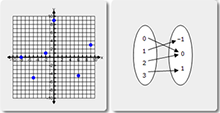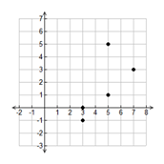Function

My Definition

Key Characteristics

The following are true of a function:

• It is a relation in which each input is associated with exactly one output.

• It can be represented with a graph, table, set of ordered pairs, or mapping diagram.

• A vertical line test can be used to determine if a graph is a function.

Example

Sort cards into two sets: cards that represent functions and cards that do not represent functions.

Function ActivityNon-examplex = y2TEKS: 8(5)(G), 8(5)(H)